## Welcome to the Second Life Forums Archive

These forums are CLOSED. Please visit the new forums HERE

# Discussion: Vertex Modelling Tool

Sanderman Cyclone
Registered User
Join date: 29 Jun 2006
Posts: 8
02-13-2007 07:10
I made this tool because I felt it would be of much help to me, or any builder making complex objects. What it does is simple, it allows you to model by positioning vertices and create fitting triangles between them. This gives u a lot of precision while building as it eliminates the need to manually position, rotate, taper and sheer prims to form a surface.

Of course this tool is only useful in certain situations, in others, prim building is more efficient, the best way is to use both methods, this tool, and traditional prim building. anyone can see that a single prim for a square is more efficient that 2 tri's.

Nevertheless, I think this tool is going to be of great help to any builder and I'm looking forward to the interesting things that could sprout from this. This tool has lot's of potential. A hint: change your way of thinking to using triangles in the most efficient ways.

I want to stress that not only me deserves credit but even more Seifert Surface, as his triangle creation scripts made this tool possible, all I've done is implemented his tri's (with permission) into this userfriendly tool.

Comments and instructions how to use can be found in the main script.

I'm giving this away to the community for free.
Feel free to do what u want with the script, just don't sell it as is.

Vertex script:
CODE
`// Vertex// by Sanderman Cyclone// also incorporates work by Seifert Surface// Jan 2007//// Use this as you want but don't resell it as is.//// How to use the Vertex Modelling Tool://// Use this tool to make triangles between preset position vectors.// This could be used for vertex modelling, as done in RL modelling apps.// This will not kill prim building, there is still the prim limit :(// Every triangle is actually a single prim so prim count could go up fast, but a good builder// keeps this in mind and creates fantastic things with this. :)// The tool works best in combination with traditional prim building, currently a limit of the tool// is that it can not make polygons, so these should be made manually unless u want to use 2 tris// for a square for example. :(//// To start vertex modelling, just rez a vertex, shift-copy it a few times, position them as you// want, and click them one by one, every time, 3 vertices are selected, a triangle will be formed,// this way you can create cool surfaces. :)//// The tool accepts 3 different voice commands over the chat on the command channel:// // 1. depth x       replace x with a float to set the depth of to be created triangles//                  I find that depth of 0.01 is good for precision while 0.1 or 1 gives crudeness.// 2. clean         removes all vertices when done building// 3. freeze        removes the scripts and triangle object from vertices, usefull if u want that //                  for some reason////// How to use the vertex modelling tool scripts:// (this is important if assembling yourself from the forums)//// Rez a prim, name it Triangle, put the Triangle script in it.// Rez a second prim, name it Vertex, put the Vertex script and the Triangle Detailer script in it.// Also put the Triangle object in the Vertex.// Resize and deform the Vertex prim to your preference. I prefer it to be a small sphere.// Your Vertex is ready to use, always keep a copy of it in inventory. Rez some Vertices in world// to start the fun. :)//// Note. you could also customize this with custom scripting or a different predefined triangle// color or whatever.//// How it works://// Basically, every time a vertex is touched, it broadcasts it's position over chat so others know// that position. These positions are saved and when an object is touched and it already has 2// positions in variable, then get third position of itself and rez a triangle between the 3 vectors.//// The triangle creation part is actually the biggest part of the system and Seifert Surface recieves// full credit for that. He gave permission on the forums for people to use his script in third party// tools.//// What I've done is integrate his triangles into a user friendly tool that will help builders with// building complex shapes. The builder should however, be aware of prim count as it could go up// fast.//integer chan = 22; //change this if u want a different command channel.vector a;vector b;vector c;vector origin;float scale = 1;float depth = 0.01;float delta = 0.01; rotation rot;integer comm_channel = -61347732;integer rez_counter;//Function for rezzing triangle between vectors a, b and c.//Credit for this goes to Seifert Surface.rez_triangle(vector a, vector b, vector c){    a = origin + scale * a;    b = origin + scale * b;    c = origin + scale * c;    //check for 2 vertices in the same place    if(llVecDist(a,b) < delta || llVecDist(b,c) < delta || llVecDist(c,a) < delta)    {  //if so, don't try to rez the triangle        return;    }    float cosA = (b - a) * (c - a);    float cosB = (c - b) * (a - b);    float cosC = (a - c) * (b - c);  //signs -ve means obtuse angle    if(cosA < 0.0)  //so the angle at a is obtuse, meaning the opposite edge must be the base of the prim    {        triangle(a, b, c, TRUE);    }    else if(cosB < 0.0)    {        triangle(b, c, a, TRUE);    }    else if(cosC < 0.0)    {        triangle(c, a, b, TRUE);    }    else //all acute angles... so we can choose which way to base the prim, so as to minimise the    {        //error introduced by the shear value having a resolution of only 0.01        float error1 = triangle(a,b,c,FALSE);        float error2 = triangle(b,c,a,FALSE);        float error3 = triangle(c,a,b,FALSE);        if(error1 < error2)        {            if(error1 < error3)            {                triangle(a,b,c,TRUE);            }            else            {                triangle(c,a,b,TRUE);            }        }        else        {            if(error2 < error3)            {                triangle(b,c,a,TRUE);            }            else            {                triangle(c,a,b,TRUE);            }        }    }}         float triangle(vector a, vector b, vector c, integer rez){        float width = llVecDist(b, c);    vector left = llVecNorm(b - c);    vector fwd = llVecNorm(left % (a - c));    vector up = fwd % left;    float height = (a - c) * up;    float y_shear = 0.5 - ((b-a) * left) / width;        if(rez)    {        vector  center = 0.5 * ( (b+c) + (height * up)  );        vector scale = <depth, width, height>;        rotation rot = llAxes2Rot(fwd, left, up);        llMessageLinked(LINK_THIS, comm_channel + rez_counter, (string)scale + "|" + (string)y_shear, NULL_KEY);                while(llVecDist(llGetPos(), center) > 9.5)        {            llSetPos(center);        }        llRezObject("Triangle", center, ZERO_VECTOR, rot, comm_channel + rez_counter);        rez_counter += 1;        return -1.0;    }    else    {        y_shear = (float)llRound(y_shear * 100.0) / 100.0;        return llVecDist(a, 0.5 * (b + c) + height * up + y_shear * width * left);        //error between where the vertex of the triangle should be and would be...    }}//function for resetting the vertexreset(){    a = ZERO_VECTOR;    b = ZERO_VECTOR;    c = ZERO_VECTOR;    llSetPrimitiveParams([PRIM_COLOR, ALL_SIDES, <1, 1, 1>, 1]);}//default statedefault{    on_rez(integer num)    {        reset();    }    state_entry()    {        llListen(chan,"","","");        llSetPrimitiveParams([PRIM_COLOR, ALL_SIDES, <1, 1, 1>, 1]);    }    touch_start(integer num_detected)    {        if(llDetectedKey(0) == llGetOwner())        {            //If clause to detect user error, resetting            if(llGetPos() == a || llGetPos() == b || llGetPos() == c)            {                llShout(chan, "reset");                reset();            }            //Else if to set vector a            else if(a == ZERO_VECTOR)            {                a = llGetPos();                llShout(chan,"vectora "+(string)a);                llSetPrimitiveParams([PRIM_COLOR, ALL_SIDES, <1, 0, 0>, 1]);            }            //Else if to set vector b            else if(b == ZERO_VECTOR)            {                b = llGetPos();                llShout(chan,"vectorb "+(string)b);                llSetPrimitiveParams([PRIM_COLOR, ALL_SIDES, <1, 0, 0>, 1]);            }            //Else if to set vector c, rez triangle, and reset            else if(c == ZERO_VECTOR)            {                c = llGetPos();                llSetPrimitiveParams([PRIM_COLOR, ALL_SIDES, <1, 0, 0>, 1]);                rez_triangle(a,b,c);                llShout(chan, "reset");                reset();            }        }    }    //listening for communication    listen(integer chan, string name, key id, string msg)    {        //checking if the speaker is owner or owner's object        if(llGetOwnerKey(id) == llGetOwner())        {            //process commands            list cmdLine = llParseString2List(msg, [" "], [""]);            string cmd = llList2String(cmdLine, 0);            cmd = llToLower(cmd);            //reset command            if(msg == "reset")            {                reset();            }            //command to set depth            else if (cmd == "depth")            {                depth = llList2Float(cmdLine, 1);            }            //freeze command, used to remove inventory from vertices            else if (cmd == "freeze")            {                llShout(chan, "freeze");                llRemoveInventory("Triangle");                llRemoveInventory("Triangle Detailer");                llRemoveInventory(llGetScriptName());             }            //clean command, to remove all vertices.            else if (cmd == "clean")            {                llShout(chan, "clean");                llDie();               }            //setting vector a from message            else if(cmd == "vectora")            {                a = (vector)llGetSubString(msg, llSubStringIndex(msg, "<"),llStringLength(msg));            }            //setting vector b from message            else if(cmd == "vectorb")            {                b = (vector)llGetSubString(msg, llSubStringIndex(msg, "<"),llStringLength(msg));            }            //setting vector c from message            else if(cmd == "vectorc")            {                c = (vector)llGetSubString(msg, llSubStringIndex(msg, "<"),llStringLength(msg));            }        }    }}`

Seifert Surface Triangle scripts, included for convenience:

Triangle:
CODE
`//Triangle Rezzee//by Seifert Surface//Nov 2006integer comm_channel = -61347732;integer comm_channel2 = 61347732;integer me;integer listen_control;integer times_to_ask = 10;default{    state_entry()    {        llListen(347, "", "", "");    }    on_rez(integer param)    {        if(param != 0)        {            me = param;            llSetObjectName((string)(me - comm_channel));            listen_control = llListen(me, "", "", "");            llShout(comm_channel2, (string)me);            llSetTimerEvent(5.0);        }    }    listen(integer chan, string name, key id, string msg)    {        if(msg == "die")        {            llDie();        }        else if(msg == "clear")        {            llRemoveInventory(llGetScriptName());           }        else if(chan == me)        {            list data = llParseStringKeepNulls(msg, ["|"], []);            vector scale = (vector)llList2String(data, 0);            if(scale.x < 0.01)            {                scale.x = 0.01;            }            if(scale.y < 0.01)            {                scale.y = 0.01;            }            if(scale.z < 0.01)            {                scale.z = 0.01;            }            float y_shear = (float)llList2String(data, 1);            llSetPrimitiveParams([PRIM_TYPE, PRIM_TYPE_BOX, 0, <0.0, 1.0, 0.0>, 0.0, <0.0, 0.0, 0.0>, <1.0, 0.0, 0.0>, <0.0, y_shear, 0.0>, PRIM_SIZE, scale]);            llSetObjectName("Object");            llRemoveInventory(llGetScriptName());   //if you want to remove scripts as soon as possible, uncomment this.            llListenRemove(listen_control);            llSetTimerEvent(0.0);        }    }    timer()    {        llShout(comm_channel2, (string)me);        times_to_ask -= 1;        if(times_to_ask <= 0)        {            llSetTimerEvent(0.0);            llSay(DEBUG_CHANNEL, "no reply after 10 tries");           }    }} `

Triangle Detailer:
CODE
`//Triangle Detailer//by Seifert Surface//Nov 2006integer comm_channel2 = 61347732;list rezzees;list tri_data;default{    state_entry()    {        llListen(comm_channel2, "", NULL_KEY, "");    }    link_message(integer sender, integer num, string str, key id)    {        rezzees += [num];        tri_data += [str];        if(llGetListLength(rezzees) > 10)        {            llSay(DEBUG_CHANNEL, "Detailer list getting long...");        }    }    listen(integer chan, string name, key id, string msg)    {        //llOwnerSay((string)llGetFreeMemory() + " " + (string)llGetListLength(rezzees));  //in case you want to see if the detailer is getting overloaded        integer who = llListFindList(rezzees, [(integer)msg]);        if(who != -1)        {            llShout((integer)msg, llList2String(tri_data, who));            rezzees = llDeleteSubList(rezzees, who, who);            tri_data = llDeleteSubList(tri_data, who, who);        }    }} `
Nada Epoch
The Librarian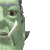Join date: 4 Nov 2002
Posts: 1,423
Original Thread
02-14-2007 11:04
/15/7e/165972/1.html
_____________________
i've got nothing.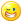Seifert Surface
Mathematician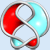Join date: 14 Jun 2005
Posts: 912
02-14-2007 11:39
Nifty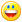_____________________
-Seifert Surface
2G!tGLf 2nLt9cG
hurly Burleigh
Registered User
Join date: 19 Sep 2005
Posts: 167
02-15-2007 09:49
This is fantastic. Will save me hours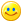)))
Sanderman Cyclone
Registered User
Join date: 29 Jun 2006
Posts: 8
02-17-2007 05:51
If anyone finds some bugs, fixes or other enhancements, feel free to post them here so I can improve it.
Chop Sands
Registered User
Join date: 21 Apr 2007
Posts: 2
05-20-2007 01:29
I must be missing somthing here,
For me, it seems to just rez a normal box prim named "Triangle" Any ideas on why this might be hapenning.